# Clausius-Mossotti relation

In physics, the Clausius-Mossotti relation   connects the relative permittivity εr of a dielectric to the polarizability α of the atoms or molecules constituting the dielectric. The relative permittivity is a bulk (macroscopic) property and polarizability is a microscopic property of matter; hence the relation bridges the gap between a directly-observable macroscopic property with a microscopic molecular property.

The Clausius-Mossotti equation for dielectric matter consisting of atoms or (non-polar) molecules is$\frac{\epsilon_r -1}{\epsilon_r+2} = \frac{1}{3\epsilon_0} N \alpha,$

where ε0 is the electric constant (permittivity of the vacuum) and N is the number density (number of atoms or molecules per volume).

The index of refraction n of a dielectric is given by$n = \sqrt{\epsilon_r \mu_r} \approx \sqrt{\epsilon_r},$

where we used that for most dielectric matter the relative magnetic permeability μr is very close to unity. Substitution of this value of n gives the Lorentz-Lorenz relation$\frac{n^2 -1}{n^2+2} = \frac{1}{3\epsilon_0} N \alpha.$

## Derivation

Consider a dielectric with number density N (number of particles per unit volume) in an external electric field E. The sum of E and an internal field Eint induces a dipole pind on each particle. The polarization vector P is the sum of the induced dipoles,$\mathbf{P} = N \mathbf{p}_\mathrm{ind}.$

From the relation between P, the electric displacement D, and the electric field E follows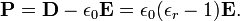$\mathbf{P} = \mathbf{D} - \epsilon_0\mathbf{E} = \epsilon_0(\epsilon_r-1) \mathbf{E}.$

To get an expression for pind, we consider a single particle (molecule or atom) in a little spherical cavity inside the dielectric. It is shown below that the total field inside this cavity can be approximated by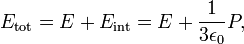$E_\mathrm{tot} = E + E_\mathrm{int} = E + \frac{1}{3\epsilon_0} P,$

where we introduced the absolute magnitudes of the vectors, which is allowed because they all lie along the z-axis, the direction of E. The field Etot is often referred to as the Lorentz local field (and in that context designated as Eloc). The Lorentz local field approximation is based on the assumption that the long-range interactions are isotropic and so it is only realistic for liquids and isotropic solids.

Now,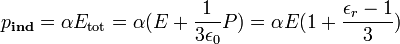$p_\mathbf{ind} =\alpha E_\mathrm{tot} = \alpha (E + \frac{1}{3\epsilon_0} P) =\alpha E (1+\frac{\epsilon_r-1}{3} )$

Further,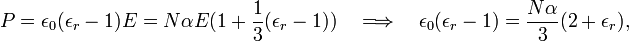$P = \epsilon_0(\epsilon_r-1) E = N\alpha E (1 + \frac{1}{3}(\epsilon_r-1)) \quad\Longrightarrow \quad \epsilon_0(\epsilon_r-1) = \frac{N\alpha}{3} (2 +\epsilon_r),$

from which the Clausius-Mossotti relation follows directly.

We neglected here the contributions to the total field in the cavity from the other molecules in the cavity. It can be shown that these contributions average out in most dielectrics.

## Local field in dielectric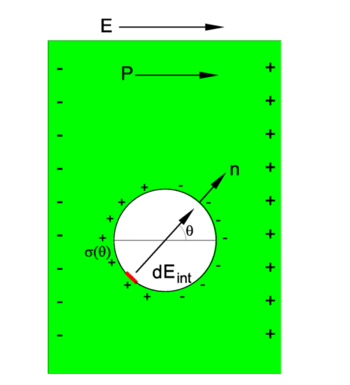Slab of dielectric (green) in external electric field E. Microscopic spherical cavity (size greatly exaggerated in drawing) of radius r in dielectric.

A macroscopic slab of dielectric is placed in an outer electric field E (the z-direction) that polarizes the dielectric, so that a charge density is created on the outer surfaces perpendicular to E. Since E "pushes" positive charge and "pulls" negative charge, the sign of the charge density on the outer surfaces is as is indicated in the figure. The polarization vector P points by definition from negative to positive charge. The surface charge densities are in absolute value equal to |P| ≡ P.

An infinitesimally small spherical cavity of radius r is made in the dielectric and inside this cavity there is vacuum with permittivity (electric constant) ε0. The cavity is so small that the polarization vector P is not affected by it. If the cavity had been rectangular with walls parallel to the charged macroscopic surfaces of the slab, the charge density on the walls of the cavity would have been the same (in absolute value) as on the outer surfaces of the slab (P), because of electric neutrality. Since the surface of the cavity is spherical, it has the charge density,$\sigma(\theta) = -\mathbf{n}\cdot\mathbf{P} = -P \cos\theta ,$

where n is a unit length vector perpendicular to the surface of the cavity at the point θ, see the figure. By definition, the positive direction of the normal n is outward.

An infinitesimal surface element at θ+π (red in the figure)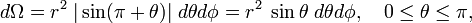$d\Omega = r^2\;|\sin(\pi+\theta)|\; d\theta d\phi=r^2\;\sin\theta\; d\theta d\phi, \quad 0\le \theta \le \pi,$

on the wall of the cavity gives a contribution to the internal electric field dEint parallel to n,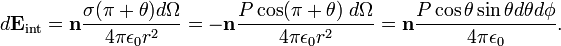$d\mathbf{E}_\mathrm{int} = \mathbf{n} \frac{\sigma(\pi+\theta) d\Omega}{4\pi \epsilon_0 r^2} = -\mathbf{n}\frac{P\cos(\pi+\theta)\; d\Omega}{4\pi \epsilon_0 r^2} =\mathbf{n} \frac{P \cos\theta\sin\theta d\theta d\phi}{4\pi \epsilon_0 }.$

The z-component of n is cosθ and hence the contribution to the field in the z direction is$dE_{\mathrm{int},z} = \frac{P}{4\pi \epsilon_0} (\cos\theta)^2\sin\theta d\theta d\phi.$

Contributions in other than the z direction cancel mutually. Integration over the whole surface gives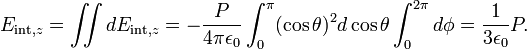$E_{\mathrm{int},z} = \iint dE_{\mathrm{int},z} = - \frac{P}{4\pi \epsilon_0} \int_{0}^{\pi} (\cos\theta)^2 d\cos\theta \int_{0}^{2\pi} d\phi = \frac{1}{3\epsilon_0} P.$

The total electric field in the cavity (the "Lorentz local field") is in the z direction and has magnitude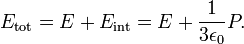$E_\mathrm{tot} = E + E_\mathrm{int} = E + \frac{1}{3\epsilon_0} P.$